##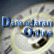Debt Ratio Regression: January 2020

Variables used in the regression

1. Debt Ratio = Debt/ (Market Value of Equity + Debt): If you can get market value of debt, use it. Else, use book value of debt.
2. Payout Ratio= Dividends/ Net Income, if Net Income is positve, not available if net income is negative.
3. Expected growth rate in EPS- next 5 years= You can use expected or even historical earnings growth, if you don't have an EPS growth forecast
4. Effective Tax Rate = Effective tax rate in most recent year

US Regression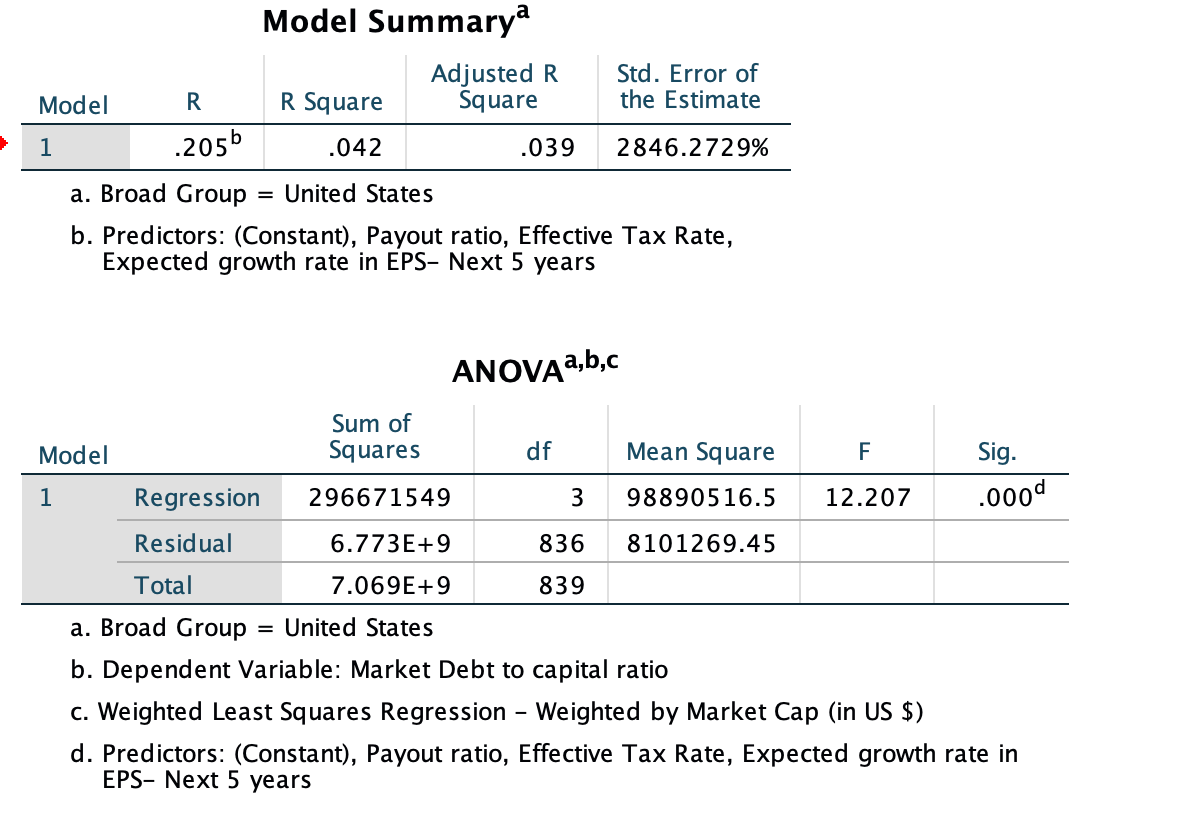## US Regression

####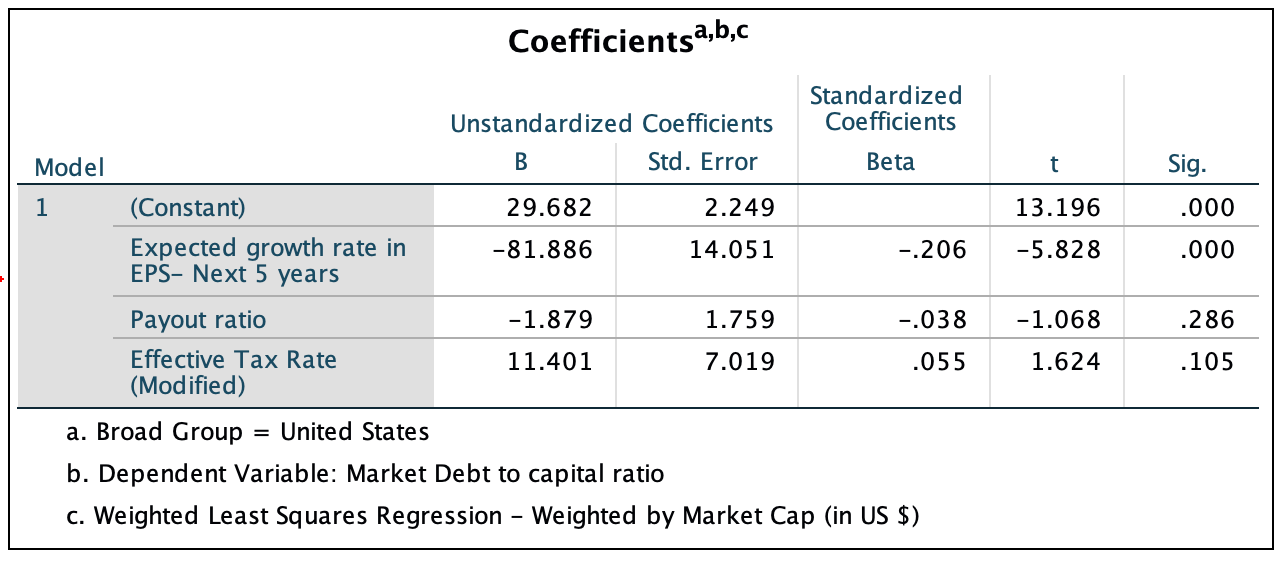Global Regression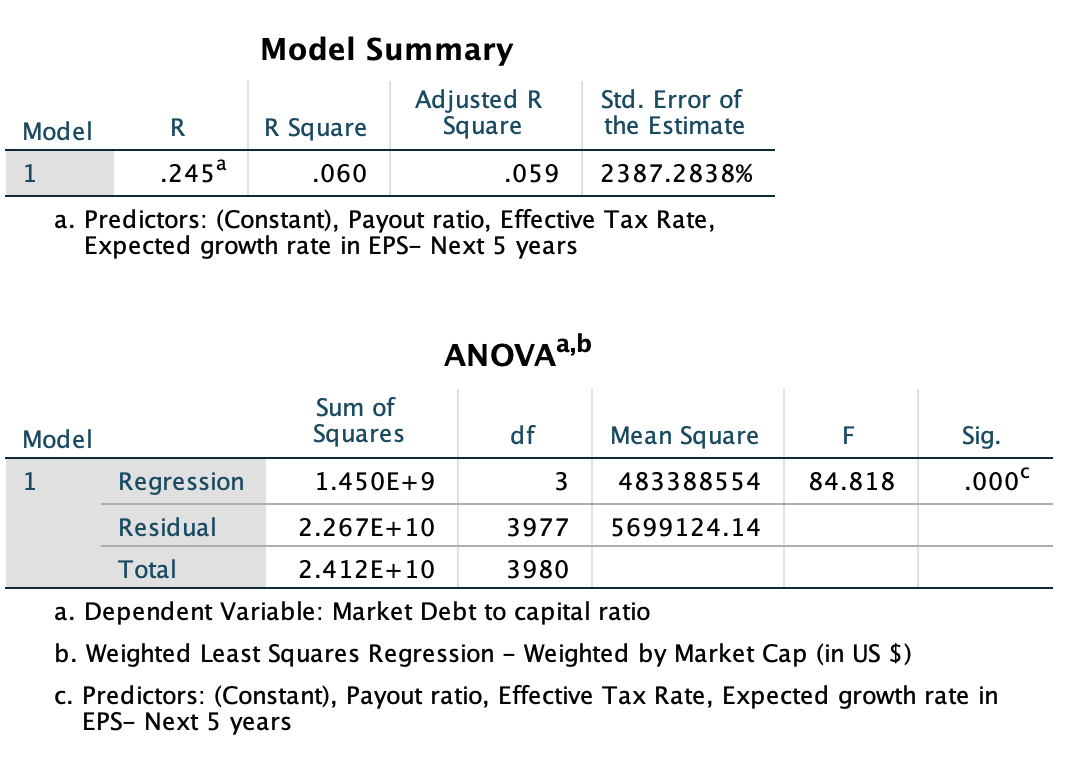## Global Regression

####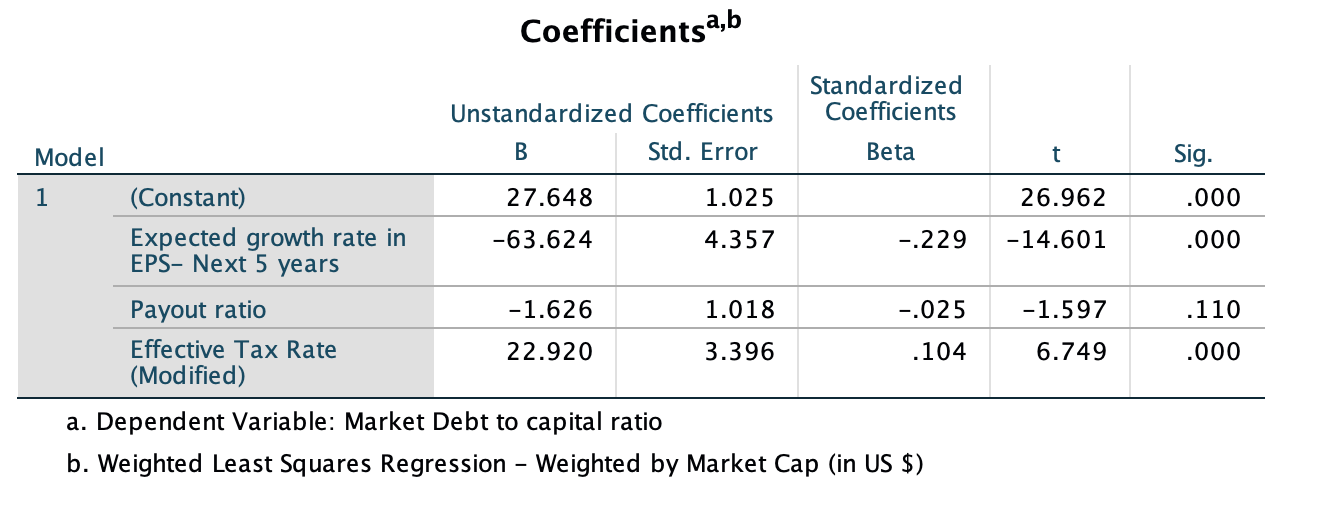• How do I use this regression?

Assume that you want to estimate the market debt ratio for a firm with the following characteristics, using the Global regression

Payout Ratio = 40%

Effective Tax Rate= 20%

Expected growth rate in EPS = 15%

• Predicted Value

Expected Debt Ratio = 27.65 +  22.92 (.20) - 63.62 (15 ) - 1.63 (40) =  22.04 or 22.04%

If your predicted value is less than zero, your predicted debt ratio is zero.